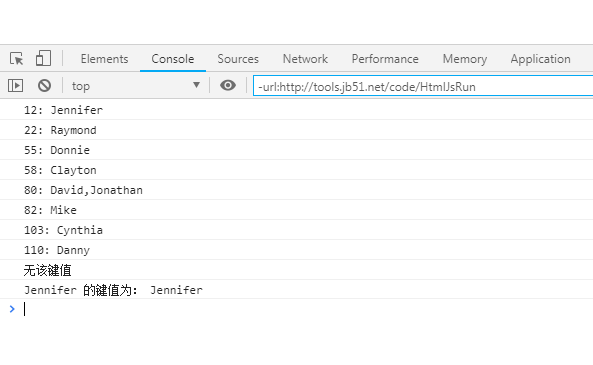# JS散列表碰撞处理、开链法、HashTable散列示例

更新时间：2019年02月08日 08:41:38   作者：白杨-M我要评论

```/**
* 散列表碰撞处理、开链法、HashTable散列。
* 将数组里的元素位置，也设置为数组，当两个数据的散列在同一个位置时，
* 就可以放在这个位置的二维数组里，解决了散列函数的碰撞处理问题
*/
function HashTable() {
this.table = new Array(137);
this.betterHash = betterHash;//散列函数
this.showDistro = showDistro;//显示散列表里的数据
this.buildChains = buildChains;//生成二维数组
this.put = put;//将数据存储到散列表
this.get = get;//从散列表中取出某个数据
}
// put for separate chaining
function put(key, data) {
var pos = this.betterHash(key);
var index = 0;
if (this.table[pos][index] == undefined) {
this.table[pos][index] = data;
}else {
while (this.table[pos][index] != undefined) {
++index;
}
this.table[pos][index] = data;
}
}
/*散列函数*/
function betterHash(string) {
const H = 37;
var total = 0;
for (var i = 0; i < string.length; ++i) {
total += H * total + string.charCodeAt(i);
}
total = total % this.table.length;
if (total < 0) {
total += this.table.length-1;
}
return parseInt(total);
}
function showDistro() {
var n = 0;
for (var i = 0; i < this.table.length; ++i) {
if (this.table[i][n] != undefined) {
console.log(i + ": " + this.table[i]);
}
}
}
function buildChains() {
for (var i = 0; i < this.table.length; ++i) {
this.table[i] = new Array();
}
}
// get for separate chaining
function get(key) {
var index = 0;
var pos = this.betterHash(key);
while ((this.table[pos][index] != undefined)&&(this.table[pos][index] != key)) {
index += 1;
}
if(this.table[pos][index] == key) {
console.log(key+" 的键值为： "+this.table[pos][index]);
return this.table[pos][index];
}else{
console.log("无该键值");
return undefined;
}
}
/*测试开链法*/
var someNames = ["David", "Jennifer", "Donnie", "Raymond",
"Cynthia", "Mike", "Clayton", "Danny", "Jonathan"];
var hTable = new HashTable();
hTable.buildChains();
for (var i = 0; i < someNames.length; ++i) {
hTable.put(someNames[i],someNames[i]);
}
hTable.showDistro();
hTable.betterHash("Jennifer");
hTable.get("Jennidfer");
hTable.get("Jennifer");

```